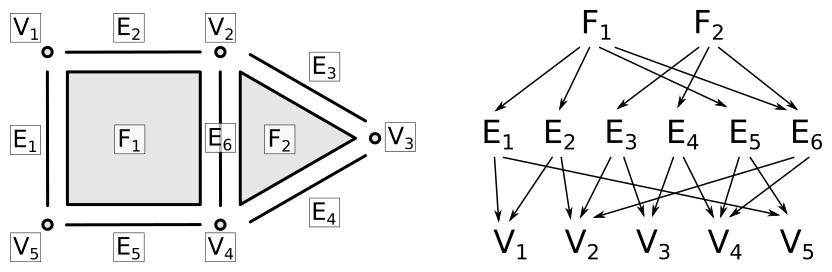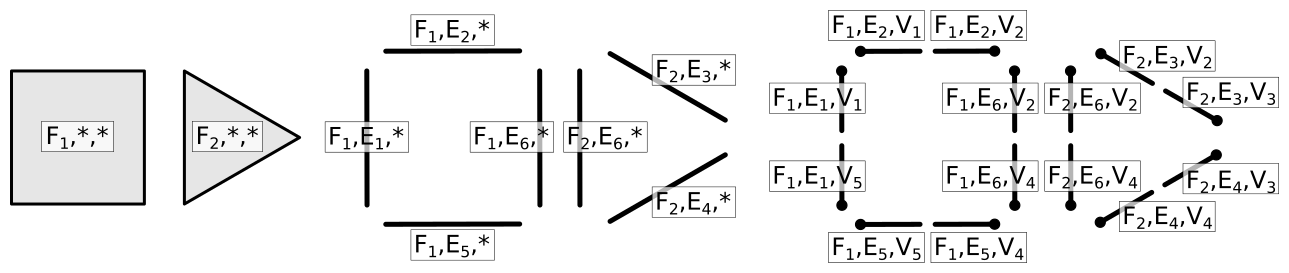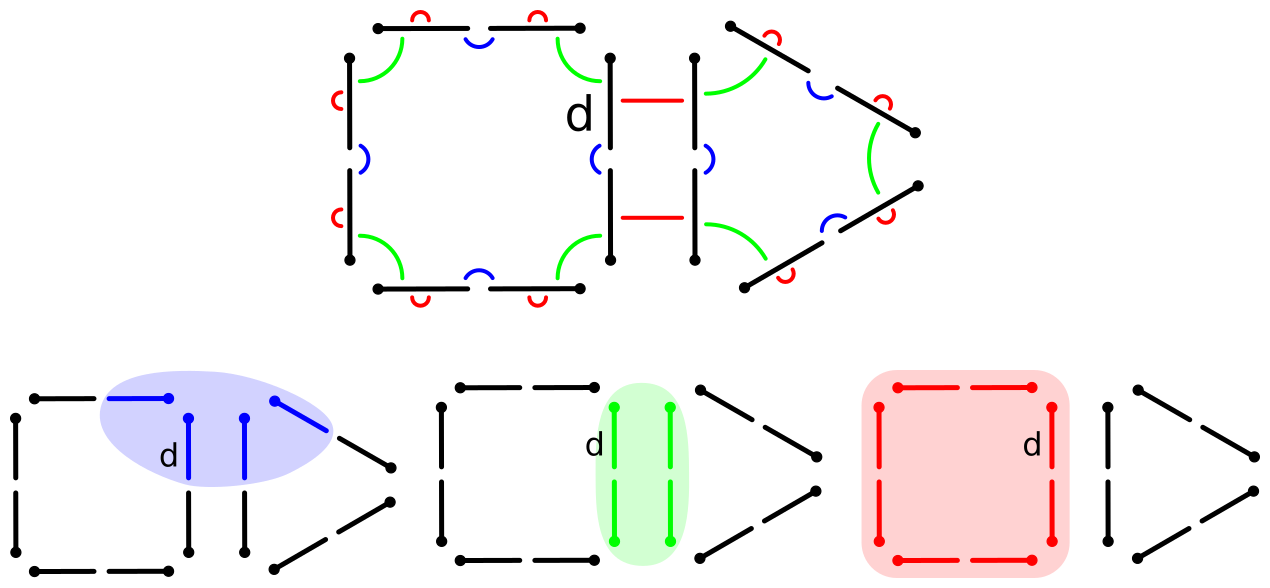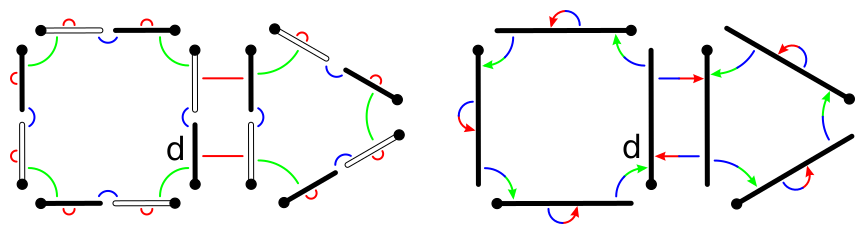View project on GitHub

# Meshes

A mesh is the cellular decomposition of geometric objects such as curves, surfaces or volumes. Mesh data structures are of fundamental importance in many fields such as surface modeling, mesh generation, finite element analysis, physical simulation, geometry processing, visualization or computational geometry.

Topological models provide neighborhood relations between the cells of the decomposition (vertices, edges, faces, volumes). This information is mandatory for many algorithms in the mentioned application fields.

The data structure should:

• provide an easy and efficient way to traverse the cells
• provide an easy and efficient way to traverse local neighborhoods
• allow to store data with the cells
• provide operators to modify the connectivity
• remain efficient both in memory & time even in complex highly dynamic settings

Many existing mesh data structures actually find their roots in the notion of combinatorial map, a mathematically defined structure that represents the subdivision of n-dimensional manifolds.

# Combinatorial maps

Combinatorial maps are dimension-independent and rely on a single element along with a simple set of relations. All the information about the cells and their incidence and adjacency relations is contained within this simple model. All neighborhood queries are resolved in optimal time (linear in the number of traversed cells) without having to maintain any additional information.

As in other topological models, there is a strong separation between the topological structure of the subdivision and the attributes that can be associated to the cells.

## From incidence graph to cell-tuples

Figure 1 shows a simple cellular decomposition of a 2-dimensional object and its incidence graph that we use as a running example in our definition.Figure 1: A cellular decomposition of a 2-dimensional object and its incidence graph

In a n-dimensional cellular decomposition, a cell-tuple is defined as an ordered sequence of cells (Cn, Cn−1, …, C1, C0) of decreasing dimensions such that ∀i, 0 < i ≤ n, Ci is incident to Ci−1. In other words, a cell-tuple corresponds to a path in the incidence graph from a n-cell to a 0-cell, i.e. a vertex. Figure 2 shows the iterative construction of all the cell-tuples generated by the cellular decomposition of Figure 1.Figure 2: Iterative construction of the cell-tuples corresponding to the cellular decomposition of Figure 1

Adjacency relations are defined on the cell-tuples: two cell-tuples are said to be i-adjacent if their path in the incidence graph share all but the i-dimensional cell. For example, (F1, E2, V1) and (F1, E2, V2) are 0-adjacent. In the context of the cellular decomposition of a quasi-manifold, it can be shown that these n+1 adjacency relations put the cell-tuples in a one-to-one relation (except for the n-adjacency at the boundary of the object where cell-tuples do not have any mate). Based on these definitions, generalized maps (or G-maps) have been proposed as a model for the representation of the cellular decomposition of n-dimensional quasi-manifolds.

## Generalized maps

Generalized maps encode a cellular decomposition with a set D of abstract elements called darts that are in one-to-one correspondance with the cell-tuples. A set of n+1 functions αi : D → D, 0 ≤ i ≤ n are defined based on the i-adjacency relations of the cell-tuples. Figure 3 shows the G-map corresponding to the cellular decomposition of the previous example. Following the previously mentioned properties of i-adjacencies on cell-tuples, αi functions are involutions, i.e. functions such that ∀d ∈ D, αi(αi(d)) = d.

Combinatorial constraints express the correct assembly of cells along their boundary. For αi functions, these constraints are expressed as follows: ∀i, j, 0 ≤ i < i + 2 ≤ j ≤ n, αi ◦ αj is an involution. If the G-map is constructed from the incidence graph of the decomposition of a quasi-manifold – a set of d-cells glued along (d-1)-cells – then those constraints are automatically satisfied.

The original cells are thus decomposed with their incidence relations into a dimension-independent set of a unique abstract entity. In order to bring back the notion of cell, the following two observations are a good starting point:

• each dart identifies a set of n cells of each dimension, i.e. those contained in the corresponding cell-tuple
• each k-cell is represented by a set of darts, i.e. all the darts whose corresponding cell-tuple contains this cell

Figure 3 illustrates these notions. Starting from the same dart d, depending on how it is interpreted, one can build the set of darts that represent its vertex, edge or face. Within each of these sets, any dart equally represents the corresponding vertex, edge or face.Figure 3: The top figure shows the G-map corresponding to the cellular decomposition of Figure 1. Darts are represented like the cell-tuples in Figure 2; α0, α1 and α2 functions are represented respectively by blue, green and red links. The three bottom figures illustrate the sets of darts representing the vertex, edge and face of dart d.

Now we only need a way to construct these sets of darts. Following the previous definitions, αi(d) is the dart that represents the same cells as d except from the i-dimensional cell. All the other αj , j = i functions will lead to darts that share the same i-cell as d. It follows that starting from a dart, the set of darts representing the same i-cell can be obtained by applying successively all the functions that maintain the i-dimensional cell unchanged, i.e. { αj, j ∈ {0, 1, …, i−1, i+1, …, n} }. Such sets of darts are formally defined as orbits, noted: < α0, …, αi−1, αi+1, …, αn >. For example in Figure 3, the vertex, edge and face of d are defined respectively by the following orbits: < α1, α2 > (d), < α0, α2 > (d) and < α0, α1 > (d).

## Oriented combinatorial maps

A G-map is able to represent orientable or non-orientable quasi-manifolds. However, a representation domain restricted to orientable objects is sufficient in many application contexts. In this case, a more compact model can be used. The orientability of a given G-map can be determined with a binary coloring process of its darts following this rule: a dart of a given color can only be linked to darts of the other color. Starting with an arbitrary dart, if the whole object can be colored this way, then the object is orientable. In this case, the darts of the G-map are partitionned in two sets D-black and D-white of equal cardinality, each one representing one of the two orientations of the object. For any dart d ∈ D, < φ1, …, φn > (d) with φi = αi ◦ α0 is the set of darts corresponding to the orientation yielded by d. For example, if d ∈ D-black, then < φ1, …, φn > (d) = D-black. Figure 4 shows the application of this process.Figure 4: Left: the darts of the G-map have been partitionned in two sets, each corresponding to one of the two orientations of the object. Right: the oriented combinatorial map yielded by dart d; φ1 = α1 ◦ α0 and φ2 = α2 ◦ α0 relations are represented respectively by blue-green and blue-red links.

As they represent the exact same object, keeping the two orientations of an orientable G-map is not necessary and one of these sets can be dropped, leading to a twice more compact representation. One orientation of an orientable G-map is actually a combinatorial map, defined as a set of darts D along with n functions φi : D → D, 1 ≤ i ≤ n, with φi = αi ◦ α0. The φ1 function is a permutation that links the ordered vertices around oriented faces. The φi, i ≤ 2 ≤ n functions are involutions, as stated by the constraint expressed above on the αi involutions. From a constructive point of view, each of these involutions allows to glue pairs of i-dimensional cells along their common (i-1)-dimensional boundary cell.

The orbits that define the cells are the following. For cells of dimension i ≥ 1, the sets of darts that represent the cells are defined by the orbit < φ1, …, φi−1, φi+1, …, φn >. Like for G-maps, starting from any dart, all the functions that maintain the i-dimensional cell unchanged are applied. For vertices, the orbit is < φ1 ◦ φ2, …, φ1 ◦ φn >.

## Embedding

Maps and G-maps only define the topology of the cellular decomposition of quasi-manifolds, i.e. the cells – represented implicitly by sets of darts – and the neighborhood relations between them. In order to consistently attach attributes to cells, any data attached to a cell has to be linked to all the darts of this cell. The most flexible solution is to associate an index to each cell. Any data associated to this index is then associated to the corresponding cell.

To model this idea, additional functions can be defined on maps. For each i, 0 ≤ i ≤ n, emb_i : D → N, is the function that associates to each dart the index of its i-dimensional cell. In order for a map to be well embedded, the following constraint must be satisfied: ∀d, d ∈ D, ∀d’ ∈ orbit_i(d), emb_i(d’) = emb_i(d). In other words, for each dimension, all the darts of the same orbit are associated to the same index.

The indexing of the cells of any dimension is completely optional. If the cells of one or even all dimensions are not indexed, the cellular decomposition and its topology are still completely defined. Indeed, cell enumeration and neighborhood traversals are performed using exclusively the darts and their relations.